# Toolbox

## Introduction

The layered structure of ggplot encourages you to design and construct graphics in a structured manner. You have learned what a layer is and how to add one to your graphic, but not what geoms and statistics are available to help you build revealing plots. This chapter lists some of the many geoms and stats included in ggplot, broken down by their purpose. This chapter will provide a good overview of the available options, but it does not describe each geom and stat in detail. For more information about individual geoms, along with many more examples illustrating their use, see the online and electronic documentation. You may also want to consult the documentation to learn more about the datasets used in this chapter.

This chapter is broken up into the following sections, each of which deals with a particular graphical challenge. This is not an exhaustive or exclusive categorisation, and there are many other possible ways to break up graphics into different categories. Each geom can be used for many different purposes, especially if you are creative. However, this breakdown should cover many common tasks and help you learn about some of the possibilities.

The examples do not go into much depth, but hopefully if you flick through this chapter, you’ll be able to see a plot that looks like the one you’re trying to create, and modify it to meet your needs.

## Overall layering strategy

It is useful to think about the purpose of each layer before it is added. In general, there are three purposes for a layer:

• To display the data. We plot the raw data for many reasons, relying on our skills at pattern detection to spot gross structure, local structure, and outliers. This layer appears on virtually every graphic. In the earliest stages of data exploration, it is often the only layer.

• To display a statistical summary of the data. As we develop and explore models of the data, it is useful to display model predictions in the context of the data. We learn from the data summaries and we evaluate the model. Showing the data helps us improve the model, and showing the model helps reveal subtleties of the data that we might otherwise miss. Summaries are usually drawn on top of the data.

If you review the examples in the preceding chapter, you’ll see many examples of plots of data with an added layer displaying a statistical summary.

• To add additional metadata, context and annotations. A metadata layer displays background context or annotations that help to give meaning to the raw data. Metadata can be useful in the background and foreground.

A map is often used as a background layer with spatial data. Background metadata should be rendered so that it doesn’t interfere with your perception of the data, so is usually displayed underneath the data and formatted so that it is minimally perceptible. That is, if you concentrate on it, you can see it with ease, but it doesn’t jump out at you when you are casually browsing the plot.

Other metadata is used to highlight important features of the data. If you have added explanatory labels to a couple of inflection points or outliers, then you want to render them so that they pop out at the viewer. In that case, you want this to be the very last layer drawn.

## Diamonds data

The `diamonds` dataset consists of price and quality information for about 54,000 diamonds. It’s built into ggplot2:

``````library(ggplot2)
dim(diamonds)
#>  53940    10
#>   carat       cut color clarity depth table price    x    y    z
#> 1  0.23     Ideal     E     SI2  61.5    55   326 3.95 3.98 2.43
#> 2  0.21   Premium     E     SI1  59.8    61   326 3.89 3.84 2.31
#> 3  0.23      Good     E     VS1  56.9    65   327 4.05 4.07 2.31
#> 4  0.29   Premium     I     VS2  62.4    58   334 4.20 4.23 2.63
#> 5  0.31      Good     J     SI2  63.3    58   335 4.34 4.35 2.75
#> 6  0.24 Very Good     J    VVS2  62.8    57   336 3.94 3.96 2.48``````

The data contains the four C’s of diamond quality, carat, cut, colour and clarity; and five physical measurements, depth, table, x, y and z, as described in Figure~.

The dataset has not been well cleaned, so as well as demonstrating interesting facts about diamonds, it also shows some data quality problems.

## Basic plot types

These geoms are the fundamental building blocks of ggplo2. They are useful in their own right, but are also used to construct more complex geoms. Most of these geoms are associated with a named plot: when that geom is used by itself in a plot, that plot has a special name.

Each of these geoms is two dimensional and requires both `x` and `y` aesthetics. All understand `colour` (or `color`) and `size` aesthetics, and the filled geoms (bar, tile and polygon) also understand `fill`. The point geom understands `shape` and the line and path geoms understand `linetype`. The geoms are used for displaying data, summaries computed elsewhere, and metadata.

• `geom_area()` draws an area plot, which is a line plot filled to the y-axis (filled lines). Multiple groups will be stacked on top of each other.

• `geom_bar(stat = "identity")` makes a barchart. We need `stat = "identity"` because the default stat automatically counts values (so is essentially a 1d geom, see displaying distributions. The identity stat leaves the data unchanged.

By default, multiple bars in the same location will be stacked on top of one another.

• `geom_line()` makes a line plot. The `group` aesthetic determines which observations are connected; see grouping for more details. `geom_path()` is similar to a `geom_line()`, but lines are connected in the order they appear in the data, not from left to right.

• `geom_point()` produces a scatterplot.

• `geom_polygon()` draws polygons, which are filled paths. Each vertex of the polygon requires a separate row in the data. It is often useful to merge a data frame of polygon coordinates with the data just prior to plotting. Drawing maps illustrates this concept in more detail for map data.

• `geom_text()` adds labels at the specified points. This is the only geom in this group that requires another aesthetic: `label`. It also has optional aesthetics `hjust` and `vjust` that control the horizontal and vertical position of the text; and `angle` which controls the rotation of the text. See specifications for more details.

• `geom_tile()` and `geom_raster()` make an image plot or level plot. The tiles form a regular tessellation of the plane and typically have the `fill` aesthetic mapped to another variable. `geom_raster()` is a faster verion of `geom_tile()` when all the tiles are the same size.

Each of these geoms is illustrated in below. Observe the different axis ranges for the bar, area and tile plots: these geoms take up space outside the range of the data, and so push the axes out.

``````df <- data.frame(
x = c(3, 1, 5),
y = c(2, 4, 6),
label = c("a","b","c")
)
p <- ggplot(df, aes(x, y, label = label)) + labs(x = NULL, y = NULL)
p + geom_point() + labs(title = "geom_point")
p + geom_bar(stat = "identity") + labs(title = "geom_bar(stat=\"identity\")")
p + geom_line() + labs(title = "geom_line")
p + geom_area() + labs(title = "geom_area")
p + geom_path() + labs(title = "geom_path")
p + geom_text() + labs(title = "geom_text")
p + geom_raster() + labs(title = "geom_raster")
p + geom_polygon() + labs(title = "geom_polygon")``````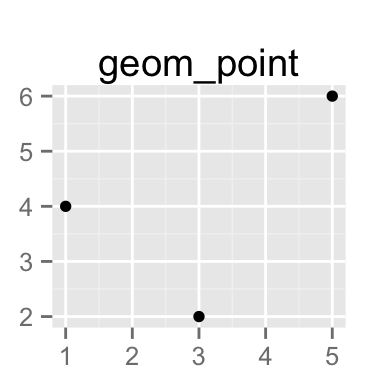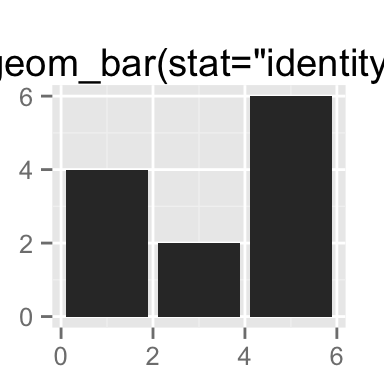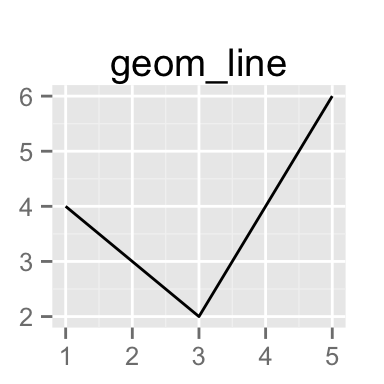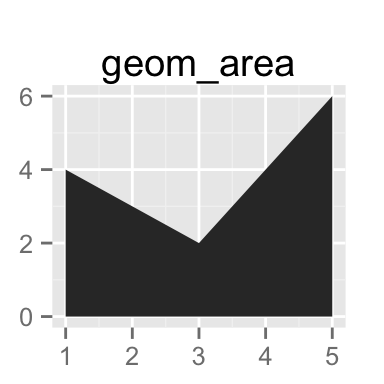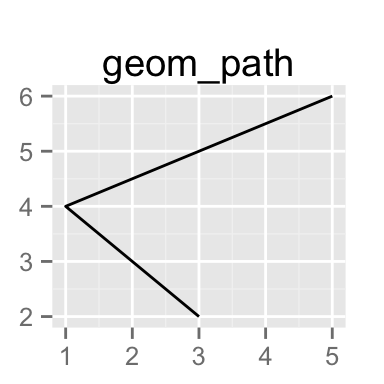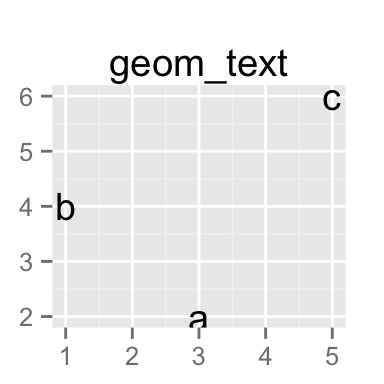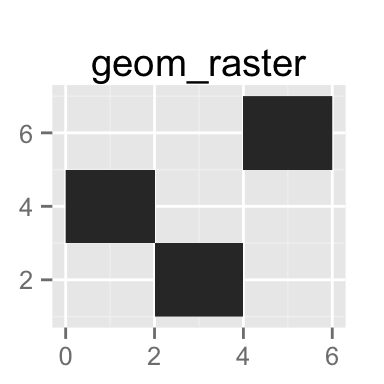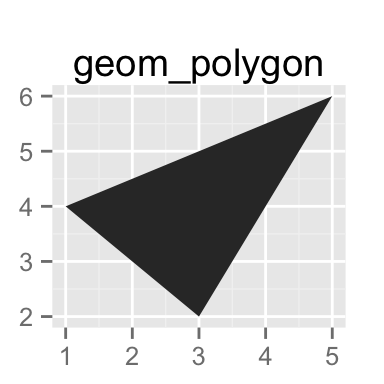### Exercises

1. What geoms would you use to draw each of the following named plots?

1. Scatterplot
2. Line chart
3. Histogram
4. Bar chart
5. Pie chart
2. What’s the different between `geom_path()` and `geom_polygon()`?

3. What low-level geoms are used to draw `geom_smooth()`? `geom_boxplot()`?

## Grouped data

Geoms can be roughly divided into individual and collective geoms. An individual geom has a distinct graphical object for each observation (row). For example, the point geom draws one point per row. A collective geoms display multiple observations with one geometric object. This may be a result of a statistical summary, like a boxplot, or may be fundamental to the display of the geom, like a polygon. Lines and paths fall somewhere in between: each line is composed of a set of straight segments, but each segment represents two points. How do we control which observations go are display in each graphical element? This is the job of the `group` aesthetic.

By default, the `group` is set to the interaction of all discrete variables in the plot. This often partitions the data correctly, but when it does not, or when no discrete variable is used in the plot, you will need to explicitly define the grouping structure, by mapping group to a variable that has a different value for each group.

There are three common cases where the default is not enough, and we will consider each one below. In the following examples, we will use a simple longitudinal dataset, `Oxboys`, from the nlme package. It records the heights (`height`) and centered ages (`age`) of 26 boys (`Subject`), measured on nine occasions (`Occasion`).

``````data(Oxboys, package = "nlme")
#>   Subject     age height Occasion
#> 1       1 -1.0000    140        1
#> 2       1 -0.7479    143        2
#> 3       1 -0.4630    145        3
#> 4       1 -0.1643    147        4
#> 5       1 -0.0027    148        5
#> 6       1  0.2466    150        6``````

### Multiple groups, one aesthetic

In many situations, you want to separate your data into groups, but render them in the same way. In other words, you want to be able to distinguish individual subjects, but not identify them. This is common in longitudinal studies with many subjects, where the plots are often descriptively called spaghetti plots. For example, the following plot shows the growth trajectory for each boy (each `Subject`):

``````ggplot(Oxboys, aes(age, height, group = Subject)) +
geom_line()``````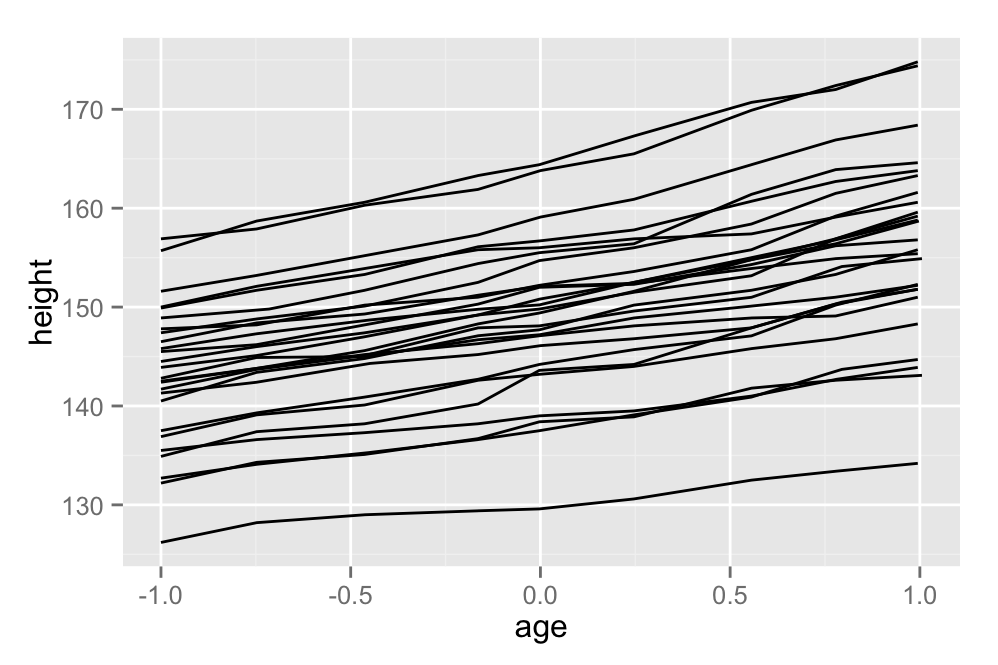If you incorrectly specify the grouping variable, you’ll get a characteristic sawtooth appearance:

``````ggplot(Oxboys, aes(age, height)) +
geom_line()``````